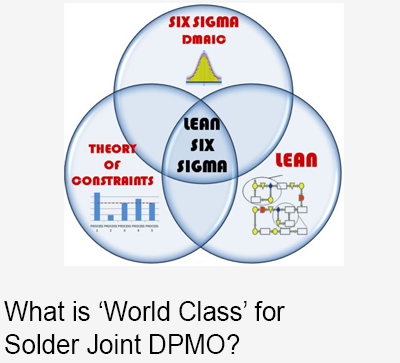A common confusion in pricing is the difference between mark-up and margin. Here's a quick explanation of both.

Mark-up is the percentage a cost is increased ("marked up") to determine a resale. For example, if an item costs \$2.00 and the mark-up is 20%, the resale is \$2.40 (the original cost plus the 20%). The simple calculation is the cost multiplied by the percentage + 1.  For example:

\$2.00 X 1.20 = \$2.40Margin, short for 'profit margin', is the percentage of a resale price that is profit. For example, if an item costs \$2.00 and the profit margin is 20%, the resale is \$2.50 (the profit of .50 is 20% of the \$2.50 resale). The simple calculation is the cost divided by 1 minus the percentage. For example:

\$2.00 / (1-.20) = \$2.50

Notice the different resales that result from applying mark-up or margin. While mark-up is common in some industries or applications, margin is by far the most commonly used pricing calculation in electronic contract manufacturing.

## SUBSCRIBE NOW